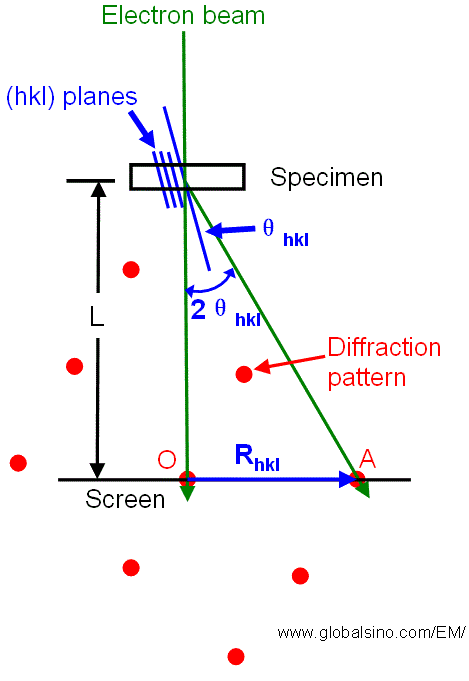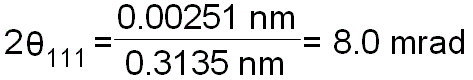Projector Lenses & Camera Lengths in TEMs/STEMs
- Practical Electron Microscopy and Database -
- An Online Book -Microanalysis | EM Book                                                                   https://www.globalsino.com/EM/

In the formation of TEM diffraction patterns as shown in Figure 3580, the reciprocal lattice spacing Rhkl, measured as the distance from the origin (O, the location of the directly transmitted beam on the screen) to some diffraction spot (A), is related to the real crystal spacing dhkl, by,

dhkl =λL/Rhkl -------------------------------- [3580a]

On the other hand, we know,

dhkl = a/(h2 + k2 + l2)1/2 -------------------- [3580b]

where,
L -- The camera length,
λ -- The wavelength of the incident electron beam,
a -- The lattice constant,
λL -- The camera constant.Figure 3580. Relationship between the reciprocal lattice spacing Rhkl and the camera length L for diffraction in a TEM.

Since λL is a constant, we can then have:
R1d1 = R2d2 = R3d3 = R4d4 = R5d5 = . . . ------------------- [3580c]

In general, based on Equation 3580a, the angles between the direct beam and diffracted beams are small so that the angles are calculated by,

2θhkl = arctan(Rhkl/L) ≈ Rhkl/L = λ/dhkl ------------------------- [3580d]

For instance, the wavelength of 200-kV TEM is 0.00251 nm, and d111 for Si is 0.3135 nm, thus the angle between direct beam and Si 111 spot is given by,------------------- [3580e]

In electron diffraction techniques (e.g. SAED and NBD), a camera length of ~500 mm is commonly used, but it can be changed depending on whether or not we want to observe the details in HOLZ. It’s quite practical that we always use a specific value of L on a particular TEM system. We may increase the L for special analysis of high-resolution diffraction, but will exclude a large number of other reflections.

In STEM and TEM systems, camera length is controlled with the projector lenses and the projector lenses can give a wide range of camera lengths for diffraction patterns. In STEMs, the projector system normally consists of not less than two lenses that provide flexibility in choosing different camera lengths and  collection angles  for imaging and spectroscopy. In many cases of HAADF imaging, the annular detection angle was practically set to be 60 - 170 mrad due to the limitation of combinations between aperture sizes and camera lengths.

 ;